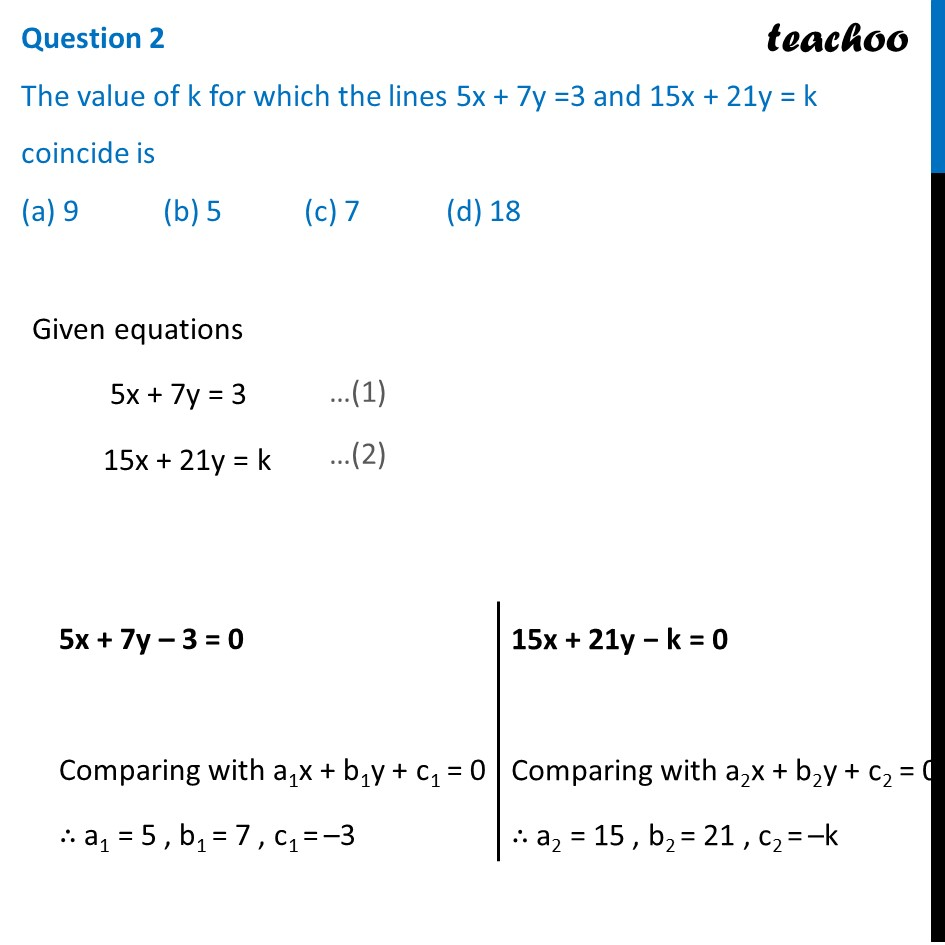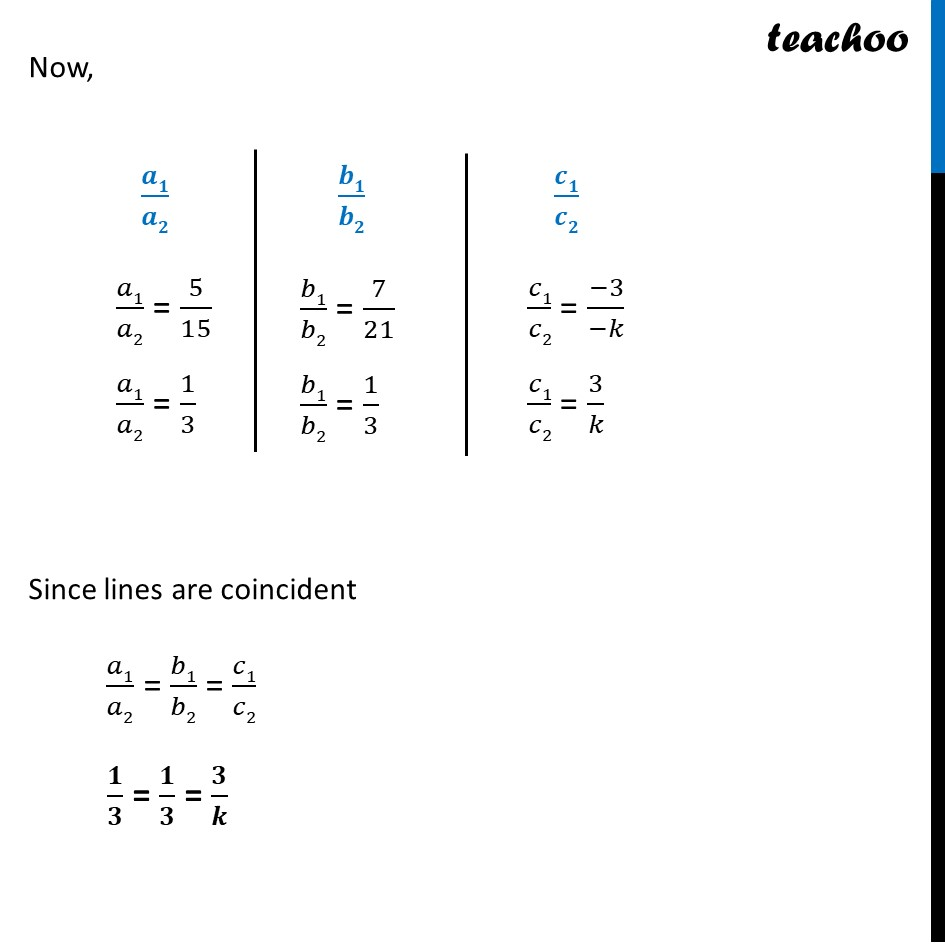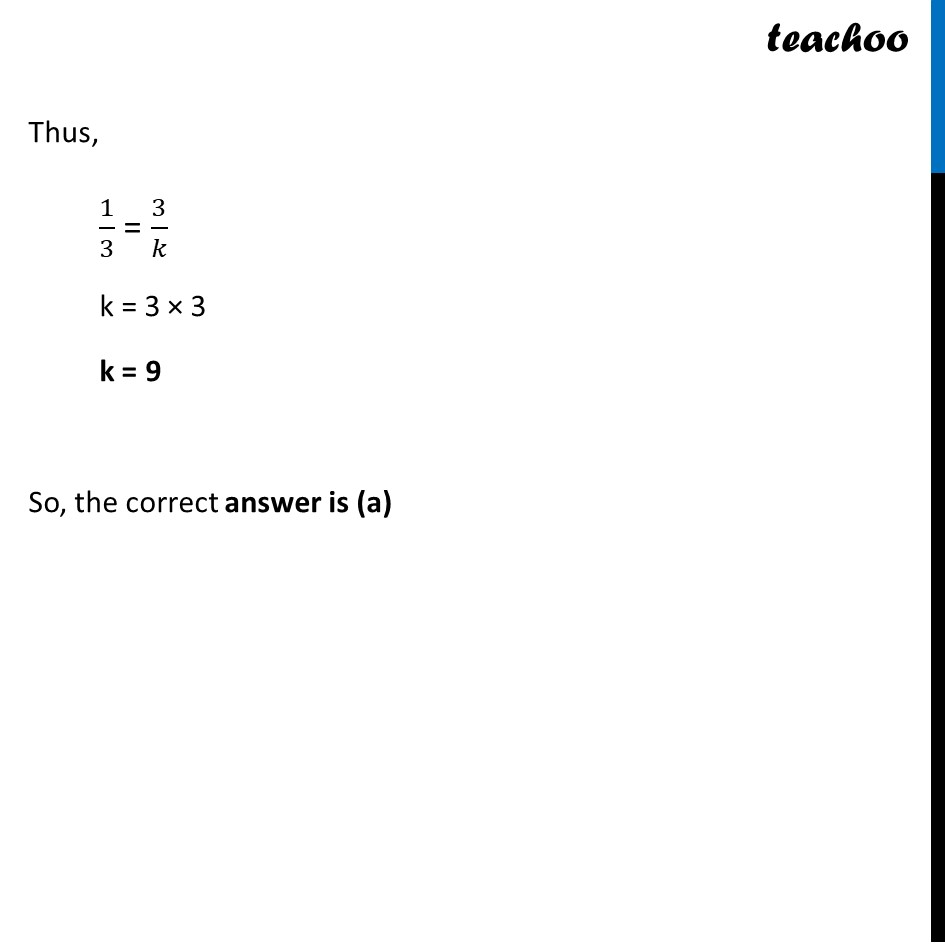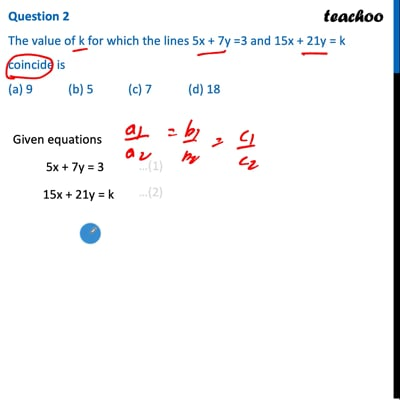CBSE Class 10 Sample Paper for 2022 Boards - Maths Standard [MCQ]

Class 10
Solutions of Sample Papers for Class 10 Boards

## (a) 9   (b) 5   (c) 7   (d) 18This video is only available for Teachoo black users

Introducing your new favourite teacher - Teachoo Black, at only ₹83 per month

### Transcript

Question 2 The value of k for which the lines 5x + 7y =3 and 15x + 21y = k coincide is (a) 9 (b) 5 (c) 7 (d) 18 Given equations 5x + 7y = 3 15x + 21y = k 5x + 7y – 3 = 0 Comparing with a1x + b1y + c1 = 0 ∴ a1 = 5 , b1 = 7 , c1 = –3 15x + 21y − k = 0 Comparing with a2x + b2y + c2 = 0 ∴ a2 = 15 , b2 = 21 , c2 = –k Now, 𝑎1/𝑎2 = 5/15 𝑎1/𝑎2 = 1/3 𝑏1/𝑏2 = 7/21 𝑏1/𝑏2 = 1/3 𝑐1/𝑐2 = (−3)/(−𝑘) 𝑐1/𝑐2 = 3/𝑘 Since lines are coincident 𝑎1/𝑎2 = 𝑏1/𝑏2 = 𝑐1/𝑐2 𝟏/𝟑 = 𝟏/𝟑 = 𝟑/𝒌 Thus, 1/3 = 3/𝑘 k = 3 × 3 k = 9 So, the correct answer is (a)